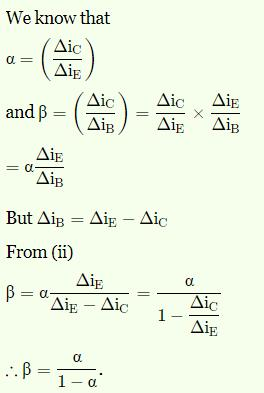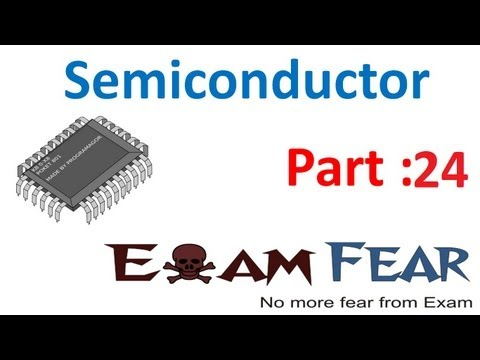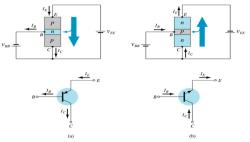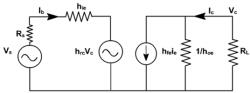Courses

Test: The Common Emitter Configuration

10 Questions MCQ Test Analog Electronics | Test: The Common Emitter Configuration

Description
Attempt Test: The Common Emitter Configuration | 10 questions in 10 minutes | Mock test for Electrical Engineering (EE) preparation | Free important questions MCQ to study Analog Electronics for Electrical Engineering (EE) Exam | Download free PDF with solutions
QUESTION: 1

The base current amplification factor β is given by_________

Solution:

The current amplification factor (β) is given by IC//IB. When no signal is applied, then the ratio of collector current to the base current is called current amplification factor of a transistor.

QUESTION: 2

In an NPN silicon transistor, α=0.995, IE=10mA and leakage current ICBO=0.5µA. Determine ICEO.

Solution:

IC=α IE +ICBO =0.995*10mA+0.5µA=9.9505mA.
IB=IE-IC=10-9.9505=0.0495mA. β=α/(1-α)=0.995/(1-0.995)=199
ICEO=9.9505-199*0.0495=0.1mA==100µA.

QUESTION: 3

A germanium transistor with α=0.98 gives a reverse saturation current ICBO=10µA in a CB configuration. When it is used in CE configuration with a base current of 0.22µA, calculate the collector current.

Solution:

Given, ICBO=10µA, α=0.98 and IB =0.22µA. IC=α/ (1-α) IB+ 1/(1-α) ICBO
0.01078+0.5=0.51078mA.

QUESTION: 4

In CE configuration, if the voltage drop across 5kΩ resistor connected in the collector circuit is 5V. Find the value of IB when β=50.

Solution:

IC=V across RL/RL=5V/5KΩ=1mA.
IB=IC/β=1/50=0.02mA.

QUESTION: 5

A transistor is connected in CE configuration. Collector supply voltage Vcc=10V, RL=800Ω, voltage drop across RL=0.8V, α=0.96. What is base current?

Solution:

Here, IC=0.8/800=1mA
β= α/ (1-α)=0.96/1-0.96=24.
Now, IB=IC/ β=1/24=41.67µA.

QUESTION: 6

The collector supply voltage for a CE configured transistor is 10V. The resistance RL=800Ω. The voltage drop across RL is 0.8V. Find the value of collector emitter voltage.

Solution:

Here, IC=0.8/800=1mA.
We know, VCE=VCC-ICRL
=10-0.8=9.2V.

QUESTION: 7

The relation between α and β is_________

Solution:QUESTION: 8

In ICEO, wt does the subscript ‘CEO’ mean?

Solution:

The subscript ‘CEO’ means that it is collector to emitter base open. It is called as the leakage current. It occurs in a reverse bias in PNP transistor. The total current can be calculated by IC=βIB+IC.

QUESTION: 9

When the signal is applied, the ratio of change of collector current to the ratio of change of base current is called_________

Solution:

The ac current gain is given by β=∆IC/∆IB. When the signal is applied, the ratio of change of collector current to the ratio of change of base current is called ac current gain.

QUESTION: 10

The range of β is _________

Solution:

Almost in all the transistors, the base current is less than 5% of the emitter current. Due to this fact, it is generally greater than 20. Usually it ranges from 20 to 500. Hence this configuration is frequently used when appreciable current gain as well as voltage gain is required.Use Code STAYHOME200 and get INR 200 additional OFF Use Coupon Code

Track your progress, build streaks, highlight & save important lessons and more!

Similar ContentRelated tests